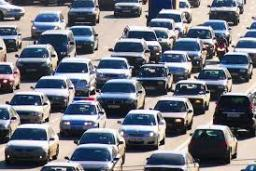# Passenger 4513

From two cities, A, and B, 210 km apart, two cars left at the same time at 8:00 AM opposite each other. A truck left place A at a speed of 60 km/h, and a passenger car left place B at 80 km/h. At what time did the cars meet?

Result

t = 9:30 hh:mm### Step-by-step explanation:

(t-8.00)·(60+80)=210

140t = 1330

t = 1330/140 = 9.5

t = 19/2 = 9.5

Our simple equation calculator calculates it.Did you find an error or inaccuracy? Feel free to write us. Thank you!

Tips for related online calculators
Do you have a linear equation or system of equations and looking for its solution? Or do you have a quadratic equation?
Do you want to convert velocity (speed) units?
Do you want to convert time units like minutes to seconds?